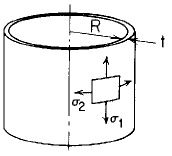Related Resources: pressure-vessel

### Cylinder Linearly Varying Radial Pressure Equation and Calculator

Cylinder Linearly Varying Radial Pressure Stress and Deflection Equation and Calculator.

Per. Roarks Formulas for Stress and Strain for membrane stresses and deformations in thin-walled pressure vessels.

 Cylindrical( R / t ) > 10 Linearly Varying Radial PressureWhen:
R / t > 10Meridional StressCircumferential or Hoop StressChange in height dimension yRotation of a meridian from its unloaded positionWhere used:
E = Modulus of Elasticity (lbs/in2)
v = Poisson's ratio
σ1,2 = stress, (lbs/in2)
t = Cylinder thickness, (in)
l = Length of cylinder, (in)
y = Length of linearly increasing load, (in)
q = Applied unit load (lbs/in2)
qo = Peak applied unit load (lbs/in2)
ψ = Rotation of a meridian from its unloaded position, positive when that meridional rotation represents an increase in ΔR when y or θ increases;

Reference:

Roarks Formulas for Stress and Strain, 7th Edition